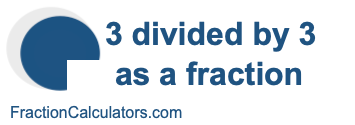3 divided by 3 as a fractionWhat is 3 divided by 3 as a fraction? Here we will show you step-by-step how to get 3 divided by 3 as a fraction.

Step 1)
In a division problem, such as 3 divided by 3 (3 ÷ 3), 3 is the dividend and 3 is the divisor. A fraction, on the other hand, has a numerator and a denominator separated by a fraction line. A division problem and a fraction are related like this:

Dividend ÷ Divisor = Numerator/Denominator

Therefore, you can convert 3 divided by 3 to a fraction like this:

3 ÷ 3 = 3/3

Step 2)
Next we will simplify the fraction, if necessary, by dividing the numerator and denominator by their greatest common divisor (GCD). This step is only necessary if the GCD is greater than 1. The GCD of 3 and 3 is 3.

3/3
(3÷3)/(3÷3)
1/1

Step 3)
Finally, we will make the fraction into a mixed fraction if the numerator is greater than or equal to the denominator (improper fraction). To convert an improper fraction to a mixed fraction, we divide the numerator by the denominator. The whole part is the whole number, and the remainder is the new numerator. The denominator stays the same.

1/1 = 1

There you have it folks. Below is 3 divided by 3 as a fraction. The answer has 3 divided by 3 as a fraction in the lowest form possible, and 3 divided by 3 as a mixed fraction, if applicable:

1/1 = 1

Division as a Fraction Calculator
3 divided by 3 as a fraction is not all we can do. You can use this tool to convert another division problem to a fraction.

÷

3 divided by 4 as a fraction
Here is the next division problem on our list that we have converted to a fraction for you.